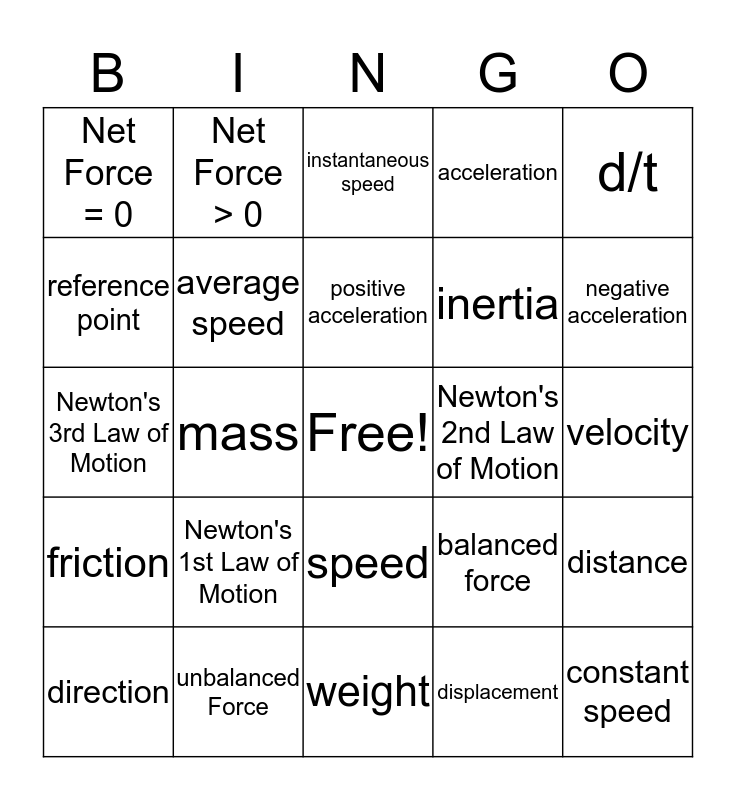# Speed/Motion ReviewThis bingo card has a free space and 24 words: Newton's 1st Law of Motion, unbalanced Force, positive acceleration, direction, d/t, Net Force = 0, reference point, Net Force > 0, Newton's 2nd Law of Motion, speed, inertia, Newton's 3rd Law of Motion, balanced force, distance, average speed, displacement, velocity, instantaneous speed, negative acceleration, mass, constant speed, friction, acceleration and weight.

## Play Online